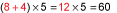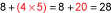## 1.2 Learn Basic Properties and Operations

This lesson will help you learn the basic properties and rules that govern math problem-solving.

### Order of Operations

When an expression has more than one operation, the order that you perform the operations could affect the answer.

 Consider this expression: $$8 + 4 \times 5$$ If you add first, the answer is 60.If you multiply first, the answer is 28.Mathematicians have agreed upon a set of rules for solving problems with more than one operation. The rules are called the order of operations.

 The Order of Operations Perform operations in parentheses or other grouping symbols. Evaluate exponents or roots. Working from left to right, perform multiplication and division steps. Working from left to right, perform addition and subtraction steps.

To remember these steps, many people memorize this acronym, or memory trick: Please Excuse My Dear Aunt Sally (PEMDAS). The letters stand for parentheses, exponents, multiplication, division, addition, and subtraction.